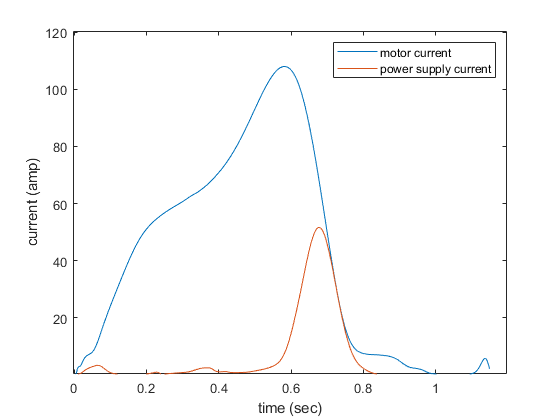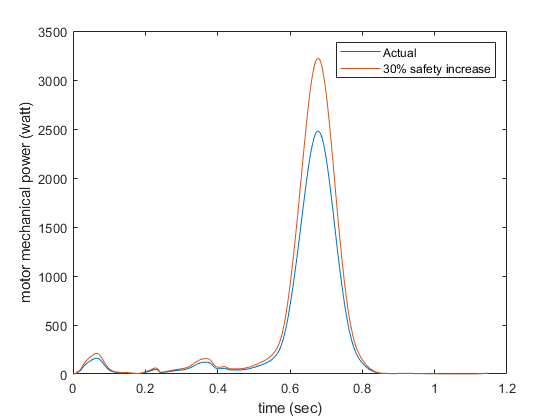# Choosing a power supply: How to account for current amplification in the controller

Hello everyone,

I know there has been many posts about power supplies but there are a few things that are not clear to me yet.

First, I am using the R100 by TMotor rated at 48V and 35 amps continuous (104 amps peak). Using the calculations given in this post (shared in another post in this forum): Things in Motion: How to select the right power source for a hobby BLDC (PMSM) motor This would mean 7800 watts. Sounds a bit excessive… Although, I understand the difference between continuous and peak operation it is critical in my application to reach peak performance for short periods of time (we are using torque profiles).

Now, what confuses me is the following from the documentation:
“The largest effect on modulation magnitude is speed. There are other smaller factors, but in general: if the motor is still it’s not unreasonable to have 50A in the motor from 5A on the power supply. When the motor is spinning close to top speed, the power supply current and the motor current will be somewhat close to each other.”

So, according to this I do not need 5000 watt power supply to reach peak current if the motor is operating at low speeds? Is this amplification happening in the controller? How can I quantify it? Is there some relationship between speed and current amplification in SVM?

Thank you!

So, according to this I do not need 5000 watt power supply to reach peak current if the motor is operating at low speeds? Is this amplification happening in the controller?

Correct on both counts, but the current amplification happens due to buck-converter action of the inverter stage + motor windings. This is the same thing as a regular switched mode power supply going from 120V 15A to 12V 150A (ideal case with no losses).

The easiest way to get a power supply requirement from system requirements is to work backwards from mechanical power. These relations are useful:

`P_mechanical = Torque * Velocity` [torque in Nm, velocity in rad/s]
`P_electrical (power suppy) = Volts * Amps`
`Torque = Iq * torque_constant` (you can dig into FOC theory, but Iq is what ODrive calls “motor current”)
`torque_constant = 8.27 / <motor KV rating>` (approximately)

For example, at 0 speed and max torque (with 35A through the windings), your motor produces zero mechanical power! The only power consumed is due to resistive losses through the windings. In theory, for a motor with zero winding resistance, power supply current would be 0! Your motor only produces/consumes 7800 Watts when it is producing max torque at max speed.

With that out of the way, you can get a good estimate of peak power supply capacity requirement if you know your maximum torque and the maximum motor speed that you need to produce that torque.

Let’s say your requirement was 2Nm at 1000rpm. I’m not sure about your motor, but one listing on the T-motor site is KV=90.
`torque_constant = 8.27 / 90 = 0.092`
`Iq = 2Nm / torque_constant = 21.7 Amps` (motor current)
`velocity [rad/s] = 1000[rev / minute] * 1/60 [minutes / second] * 2pi [radians / turn] = 104.7 rad/s`
`P_mechanical = torque * velocity = 2Nm * 104.7rad/s = 209.4 Watts`
`P_electrical = P_mechanical = 209.4 Watts`
`P_electrical / 48V = 4.36 Amps` (power supply current)

So as you can see, using conservation of power, power supply current != motor current. This ignores losses, so it would be safe to bump this power requirement figure by 20 to 30% to avoid calculating losses precisely.

3 Likes

Hello Patrick,

Thank you for the detail response. It was quite informative!
I am sharing a few calculations I made using this procedure. According to this a 48V 3500Watt supply to account for losses would be sufficient to reach peak performanceThanks!1 LikeBonjour ! s’il vous plait tu peux nous dire comment tu as pu faire le graphe pour la puissance ?